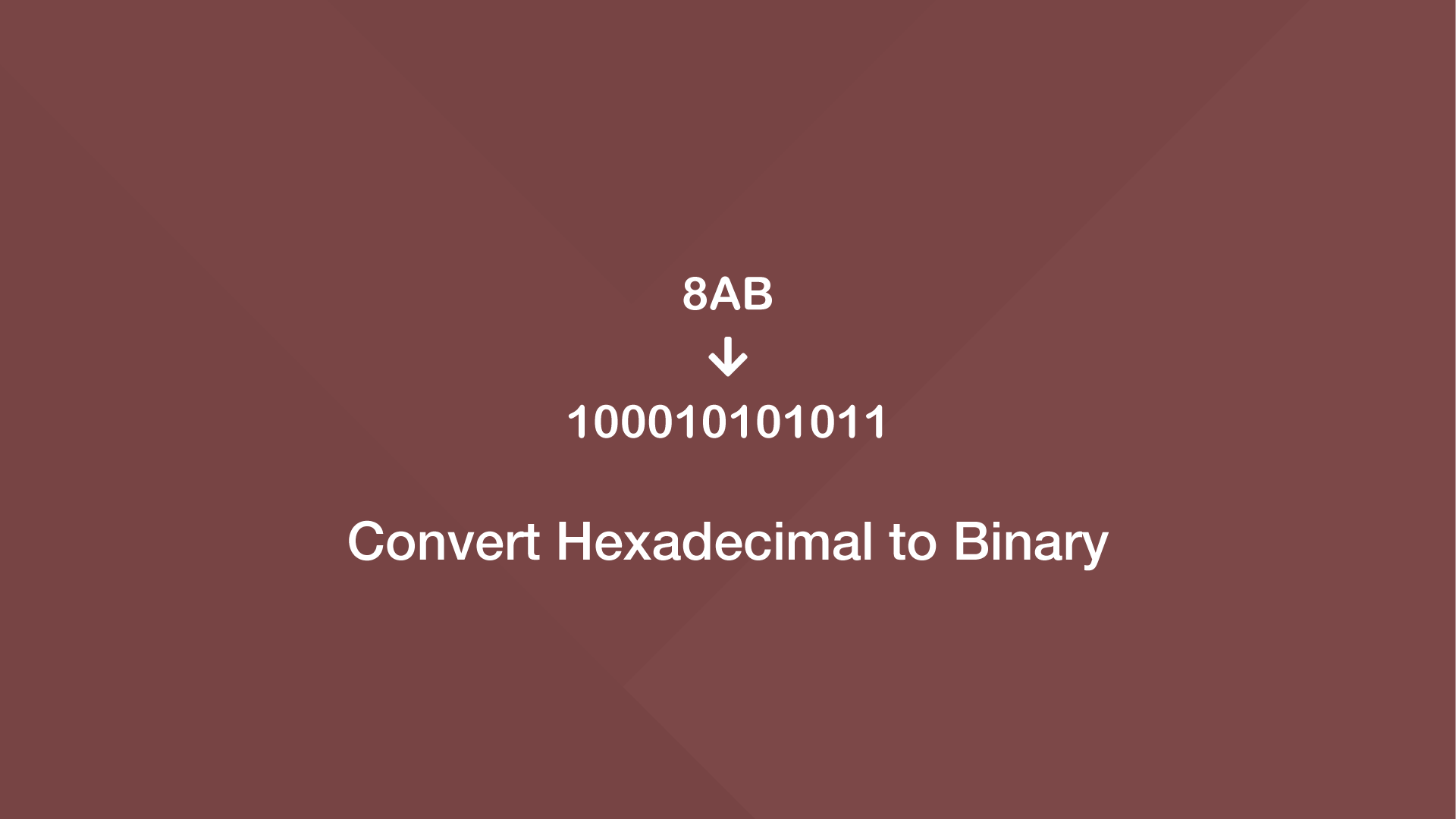# How to Convert Hexadecimal to Binary in PythonTo convert hexadecimal to binary form, first, convert it to a decimal with the `int()` function, then use the Python `bin()` function to get binary from the decimal.

## Hex to Bin using the Python int() Function

To demonstrate this, let's convert a hex value into its equivalent representation in binary.

``````result = bin(int('8AB', 16))

print(result)
``````
``````0b100010101011
``````

If you don't need the binary to be prefixed with `0b`, trim the first two characters like this:

``````result = bin(int('8AB', 16))

trimmed_res = result[2:]

print(trimmed_res)
``````
``````100010101011
``````

Essentially we are accessing everything after the second index then storing it in a new variable.

## Hex to Bin using the Python literal_eval() Function

You can also use the `literal_eval()` function to convert hex to binary. The one difference is you'll need to prefix the hexadecimal with `0x` so the parser can understand it.

``````from ast import literal_eval

result = bin(literal_eval('0x8AB'))

print(result[2:])
``````
``````100010101011
``````

#### Related Tutorials### How to Convert a String to a Number in JavaScript

August 20, 2020### Convert Int to Binary in Python

November 24, 2021### Convert Hexadecimal to Decimal in Python

November 22, 2021### How to Add Two Hex Numbers in Python

November 16, 2021### How to Convert a String to Hex in Python

April 07, 2021July 15, 2021